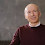## Tuesday, March 28, 2017

### Parity Games in Quasipolynomial Time

In one of the hallway discussions of last week's Dagstuhl I learned about an upcoming STOC paper Deciding Parity Games in Quasipolynomial Time by Cristian Calude, Sanjay Jain, Bakhadyr Khoussainov, Wei Li and Frank Stephan. Hugo Gimbert and Rasmus Ibsen-Jensen offer a simplified proof of the correctness of the algorithm.

A Parity Game works as follows: An instance is a finite directed graph where every vertex has at least one outgoing edge, integer weights on the vertices and a designated starting vertex. Alice and Bob take turns choosing the next vertex by following an edge from the current vertex. They play this game infinitely long and Alice wins if the the largest weight seen infinitely often is even. Not trivial to show but the game is determined and memoryless, no matter the graph some player has a winning strategy, and that strategy depends only the current vertex and not the history so far. That puts the problem into NP∩co-NP and unlikely to be NP-complete.

Like graph isomorphism, whether there exists a polynomial-time algorithm to determine the winner of a parity game remains open. Also like graph isomorphism we now have a quasipolynomial-time (exponential in logk) algorithm, an exponential improvement. Parity games have some applications to verification and model checking and some at Dagstuhl claim the problem is more important than graph isomorphism.

One difference: If you had to guess who would make the big breakthrough in graph isomorphism, László Babai would be at the top of your list. But many of the authors of this new parity games paper, like Frank Stephan and Sanjay Jain, focus mostly on computability and rarely worry about time bounds. Their algorithm does have the flavor of a priority argument often found in computability theory results. A nice crossover paper.

1.2.3.